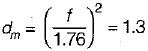Courses

# Test: Sediment Transport & Design of Irrigation Channel - 2

## 10 Questions MCQ Test GATE Civil Engineering (CE) 2022 Mock Test Series | Test: Sediment Transport & Design of Irrigation Channel - 2

Description
This mock test of Test: Sediment Transport & Design of Irrigation Channel - 2 for Civil Engineering (CE) helps you for every Civil Engineering (CE) entrance exam. This contains 10 Multiple Choice Questions for Civil Engineering (CE) Test: Sediment Transport & Design of Irrigation Channel - 2 (mcq) to study with solutions a complete question bank. The solved questions answers in this Test: Sediment Transport & Design of Irrigation Channel - 2 quiz give you a good mix of easy questions and tough questions. Civil Engineering (CE) students definitely take this Test: Sediment Transport & Design of Irrigation Channel - 2 exercise for a better result in the exam. You can find other Test: Sediment Transport & Design of Irrigation Channel - 2 extra questions, long questions & short questions for Civil Engineering (CE) on EduRev as well by searching above.
QUESTION: 1

### A stable channel has three degrees of freedom in the sense that the depth, width and slope can adjust to the given discharge and sediment conditions. In this sense which of the following methods of canal design gives a true regime channel for silt laden flow in fine alluvium?

Solution:

According to Lacey’s theory the dimensions of bed width, depth and slope of canal attain a state of equilibrium with time which is called true regime state. Lacey defined a regime channel as a stable channel transporting a minimum bed load consistent with fully active bed.

QUESTION: 2

Solution:
QUESTION: 3

### Lacey, in his regime equations, has adjusted his silt factor to be unity for the standard silt used by Kennedy. It is known that Kennedy used Bari-Doab alluvium as the standard silt in his equation. Then, the median size, in mm, of Bari-Doab alluvium and the critical velocity ratio for this silt are, respectively

Solution:
QUESTION: 4

Average bed material load of a regime channel conforming to Lacey equations will be of the order of

Solution:
QUESTION: 5

The total number of independent equations that form the Lacey's regime theory is

Solution:

Lacey collected a large number of data of stable channels in Indo-Ganetic plains. Analyzing the data he gave the following equations of regime channel relating regime velocity Vc, silt factor f, hydraulic radius R, area A, sediment size in mm and bed slope S,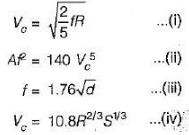These four equations are the Lacey’s basic regime flow equations.

QUESTION: 6

The velocity of flow in a channel with a depth of 1 mt calculated by Kennedy’s theory with a critical velocity ratio as 1.2 is

Solution: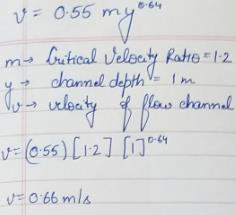QUESTION: 7

A regime channel has a discharge of 100 m3/s. It will have a perimeter of

Solution: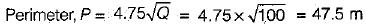QUESTION: 8

When an alluvial channel attains its regime it will have side slopes

Solution:
QUESTION: 9

For medium silt whose average grain size is 0,16 mm, Lacey’s silt factor is likely to be

Solution:

Lacey’s silt factor,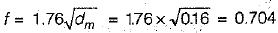QUESTION: 10

The Lacey’s silt factor for a particular alluvium is 2.0. This alluvium would comprise

Solution:

Lacey’s silt factor,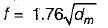∴